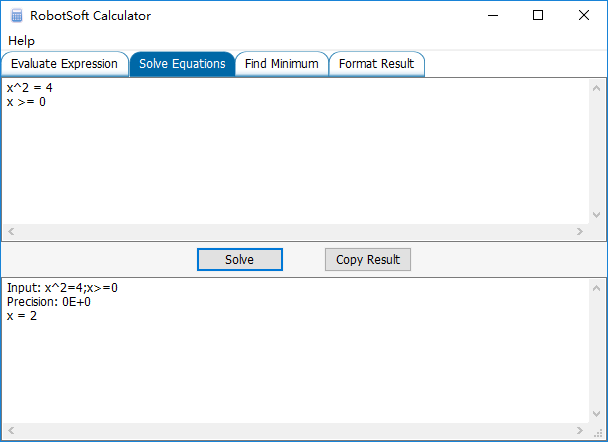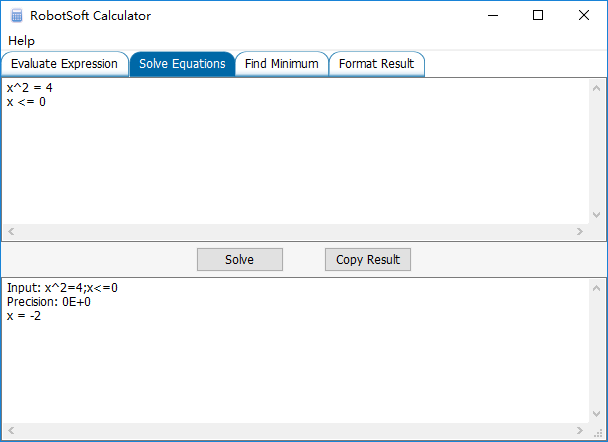# RobotSoft Calculator now supports Greater Equal and Less Equal command

Nov 192016

The Solve Equations feature of RobotSoft Calculator now supports Greater Equal and Less Equal command. Different conditions may get different results.

Assume x >= 0:Assume x <= 0:Sorry, the comment form is closed at this time.

 © 2022 RobotSoft Suffusion theme by Sayontan Sinha